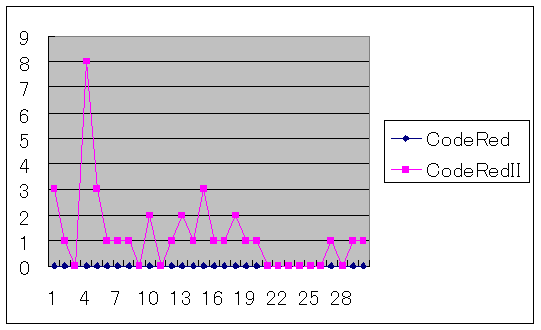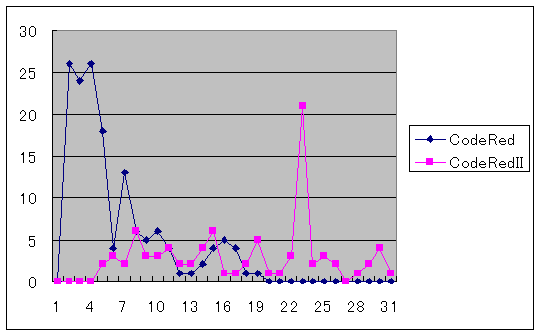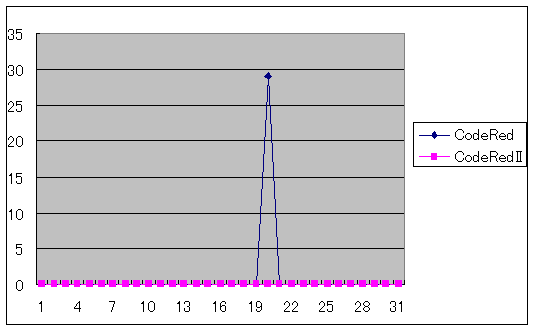YAMAHA RT\$B%7%j!<%:\$K4XO"\$9\$kOCBj(B

CodeRed\$B\$K4X\$9\$k8x3+>pJs(B

 \$B?75,:n@.F|(B 2001/Aug/27 \$B:G=*JQ99F|(B 2018/Nov/06 \$BJ8=q%5%\$%:(B 13KB

CodeRed\$B\$K4X\$9\$k8x3+>pJs(B

• CodeRed\$B\$+\$i\$N(BHTTP\$B\$NMW5a%a%C%;!<%8FbMF(B (\$BI=<(\$N\$?\$a(B50\$BJ8;z\$G2~9T(B)  ```GET /default.ida?NNNNNNNNNNNNNNNNNNNNNNNNNNNNNNNNN NNNNNNNNNNNNNNNNNNNNNNNNNNNNNNNNNNNNNNNNNNNNNNNNNN NNNNNNNNNNNNNNNNNNNNNNNNNNNNNNNNNNNNNNNNNNNNNNNNNN NNNNNNNNNNNNNNNNNNNNNNNNNNNNNNNNNNNNNNNNNNNNNNNNNN NNNNNNNNNNNNNNNNNNNNNNNNNNNNNNNNNNNNNNNNN%u9090%u6 858%ucbd3%u7801%u9090%u6858%ucbd3%u7801%u9090%u685 8%ucbd3%u7801%u9090%u9090%u8190%u00c3%u0003%u8b00% u531b%u53ff%u0078%u0000%u00=a HTTP/1.0 ```
• CodeRedII\$B\$+\$i\$N(BHTTP\$B\$NMW5a%a%C%;!<%8FbMF(B (\$BI=<(\$N\$?\$a(B50\$BJ8;z\$G2~9T(B)  ```GET /default.ida?XXXXXXXXXXXXXXXXXXXXXXXXXXXXXXXXX XXXXXXXXXXXXXXXXXXXXXXXXXXXXXXXXXXXXXXXXXXXXXXXXXX XXXXXXXXXXXXXXXXXXXXXXXXXXXXXXXXXXXXXXXXXXXXXXXXXX XXXXXXXXXXXXXXXXXXXXXXXXXXXXXXXXXXXXXXXXXXXXXXXXXX XXXXXXXXXXXXXXXXXXXXXXXXXXXXXXXXXXXXXXXXX%u9090%u6 858%ucbd3%u7801%u9090%u6858%ucbd3%u7801%u9090%u685 8%ucbd3%u7801%u9090%u9090%u8190%u00c3%u0003%u8b00% u531b%u53ff%u0078%u0000%u00=a HTTP/1.0 ```

• \$B=87WFbMF\$K\$D\$\$\$F(B

www.rtpro.yamaha.co.jp\$B\$NB-@W(B\$B\$N%Q%?!<%s\$N(B \$B!V\$"\$k\$R\$H\$D\$N%0%m!<%P%k(BIP\$B%"%I%l%9(B(www.rtpro.yamaha.co.jp)\$B\$rBP>]\$H\$7\$?(BCodeRed\$B\$NA}?#3hF0!W(B \$B\$r=87W\$7\$^\$7\$?!#(B

CodeRedII\$B\$O!"(BNimda(\$B%K%`%@(B)\$B\$KCV\$-Be\$k\$N\$G\$O\$J\$\$\$+\$H\$b(B \$B;W\$(\$k\$N\$G\$9\$,!"(B2001\$BG/(B10\$B7n(B1\$BF|\$NCJ3,\$G\$bA}?"3hF0\$r\$7\$F\$\$\$k(B \$B5\$G[\$,\$"\$j\$^\$9!#(B
Nimda\$B\$J\$I\$r4^\$a\$^\$7\$F!"?H\$N2s\$j\$N:F3NG'\$r\$*4j\$\$\$7\$^\$9!#(B

• 2001\$BG/(B9\$B7n\$N%"%/%;%97o?t(B(\$B=D<4(B:\$B7o?t(B,\$B2#<4(B:\$BF|(B)• 2001\$BG/(B8\$B7n\$N%"%/%;%97o?t(B(\$B=D<4(B:\$B7o?t(B,\$B2#<4(B:\$BF|(B)• 2001\$BG/(B7\$B7n\$N%"%/%;%97o?t(B(\$B=D<4(B:\$B7o?t(B,\$B2#<4(B:\$BF|(B)• \$B%k!<%?\$h\$j\$b!"\$^\$:!"(BIIS\$B!#(B

CodeRed\$B\$O!"(BIIS\$B\$rA@\$C\$?%o!<%`\$G\$9!#(B
\$BNc\$(!"(BCodeRed\$B\$,?/F~\$7\$F\$b!"(BIIS\$B\$KE,@Z\$J=hCV\$,\$5\$l\$F\$\$\$l\$P!"(B \$B%\$%s%H%i%M%C%HFb\$NB;32\$dBh;0 IIS\$B\$r;H\$C\$F(BWWW\$B%5!<%P\$rN)\$A>e\$2\$F\$\$\$kJ}\$O!"I,\$:!"3NG'!u=hCV\$7\$F\$/\$@\$5\$\$!#(B

\$B"*(B\$B%^%\$%/%m%=%U%HpJs(B

• WWW\$B%5!<%P5!G=\$r;}\$C\$?%k!<%?(B

RT80i\$B!"\$*\$h\$S(BRTA50i\$B\$N=i4|%j%S%8%g%s\$N(BWWW\$B%5!<%P5!G=\$K\$O!"(B \$B!V(BWWW\$B%5!<%P5!G=\$N@HpJs(B\$B!W\$NLdBj\$,\$"\$j!"(B CodeRed\$B\$NA}?#3hF0\$NBP>]\$K\$J\$C\$?\$H\$-!"%k!<%?\$,%j%9%?!<%H\$9\$k\$J\$I(B \$B\$NLdBj\$,H/@8\$9\$k\$3\$H\$,\$"\$j\$^\$9!#(B
\$B3:Ev\$9\$k5!WWW\$B%5!<%P5!G=\$N@HpJs(B\$B!W\$G;XDj\$5\$l\$?%U%!!<%`%&%'%"\$X%j%S%8%g%s%"%C%W(B(\$B99?7(B)\$B\$9\$k\$3\$H\$r\$*A&\$aCW\$7\$^\$9!#(B

\$B"*(BWWW\$B%5!<%P5!G=\$N@HpJs(B

• CodeRed\$B\$,A}?#\$7\$F\$\$\$k;~\$N%M%C%H%o!<%/(B

• CodeRed\$B\$NA}?#3hF0\$,3hH/\$K\$J\$C\$F\$\$\$k%M%C%H%o!<%/\$O!"(B \$BHs>o\$K9bIi2Y\$K\$J\$j!"@5>o\$JDL?.\$,\$G\$-\$J\$/\$J\$k>l9g\$,(B \$B\$"\$j\$^\$9!#(B
• \$B%"%I%l%9JQ495!G=(B(NAT\$B\$d(BIP\$B%^%9%+%l!<%I(B)\$B\$N4IM}%F!<%V%k\$,(B \$B0n\$l\$k\$3\$H\$K\$h\$j!"DL?.ITG=\$K\$J\$k\$J\$I\$N2DG=@-\$b9M\$(\$i\$l\$^\$9!#(B
• \$B<+%M%C%H%o!<%/\$,!"30It\$N(BCodeRed\$B\$,A}?"3hF0\$NBP>]\$H\$5\$l\$F\$7\$^\$C\$?>l9g!"(B \$B967b\$,7c\$7\$\$(B(\$B5^B.\$KA}?"\$7\$F\$\$\$k(B)\$B>l9g\$K\$O!"(BCodeRed\$B\$K\$h\$k%H%i%U%#%C%/\$G!"(B \$B2s@~\$dDL?.5!4o\$NIi2Y\$,9b\$/\$J\$j!"%Q%s%/>uBV\$K\$J\$k>l9g\$b\$"\$j\$^\$9!#(B

• \$B%\$%s%?!<%M%C%H@\B3MQ%k!<%?(B

CodeRed\$B\$,F~\$i\$J\$\$\$h\$&\$K8MDy\$j\$r87=E\$K\$7\$F\$*\$/\$3\$H\$b=EMW\$G\$9\$,!"(B \$BK|\$,0l<+%M%C%H%o!<%/\$,HH\$5\$l\$?>l9g\$K(BCodeRed\$B\$,30It\$K4m32\$rM?\$(\$J\$\$(B \$B\$h\$&\$J=hCV\$b\$"\$k\$HNI\$\$\$G\$9\$M!#(B

\$BBP:vNc(B

1. \$B%\$%s%?!<%M%C%H@\B3MQ%k!<%?\$G(BIIS\$B\$+\$i%\$%s%?!<%M%C%H\$X\$N(B HTTP\$B@\B3\$r%U%#%k%?\$G6X;_\$7\$F\$*\$/!#(B(SYN\$B%Q%1%C%H\$KCmL\(B)
2. \$B%-%c%C%7%e%5!<%P\$d%U%!%\$%"%&%)!<%k\$J\$I\$K(BCodeRed\$BBP:v5!G=\$,(B \$B\$"\$l\$P!"MxMQ\$9\$k!#(B

• \$B%\$%s%?!<%M%C%H@\B3MQ%k!<%?\$G!"(BIIS\$BH/\$N(BSYN\$B%Q%1%C%H\$rGK4~\$9\$k%U%#%k%?Nc(B

\$B@_Dj\$NJ}?K(B:
\$B8x3+(BWWW\$B%5!<%P(B(IIS,192.168.0.2)\$B\$+\$i%k!<%?\$r7PM3\$7\$FB>\$N(BWWW\$B%5!<%P\$X%"%/%;%9\$9\$k(B \$B\$3\$H\$r6X;_\$9\$k!#(BTCP\$B\$N(Bsyn\$B%Q%1%C%H\$r%k!<%?\$N(BLAN\$BB&\$GGK4~\$9\$k!#(B

\$B8x3+(BWWW\$B%5!<%P(B(IIS,192.168.0.2)\$B\$N(BWWW\$B%5!<%P\$X\$N@\B3MW5a\$N%Q%1%C%H\$r%k!<%?\$N(BLAN\$BB&\$GGK4~\$9(B \$B\$k%U%#%k%?5-=RNc(B  ```ip filter 1 pass 192.168.0.2 * established www * ip filter 2 reject 192.168.0.2 * tcp www * ip filter 3 pass * * * * * ``` ```ip lan secure filter in 1 2 3 ```

tcpflag\$B5!G=\$rl9g\$N%U%#%k%?5-=RNc(B

 ```ip filter 1 reject 192.168.0.2 * tcpflag=0x0002/0x00ff www * ip filter 2 pass * * * * * ``` ```ip lan secure filter in 1 2 ```

[ FAQ for RT-Series ]
[ FAQ for TOPIC / files ]